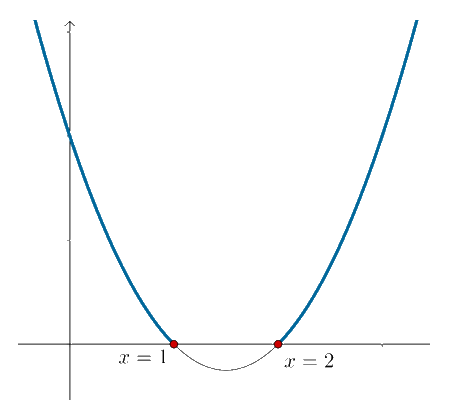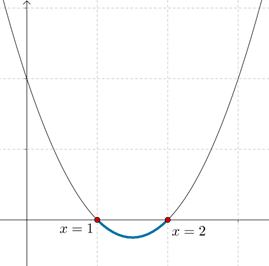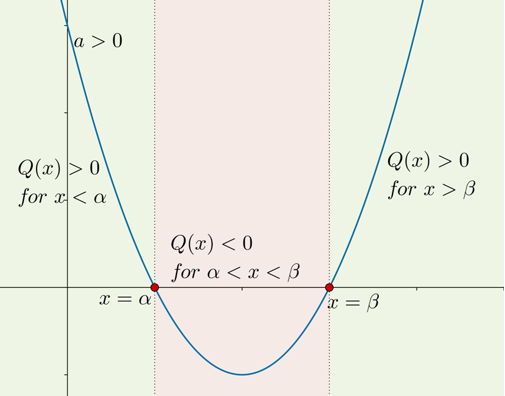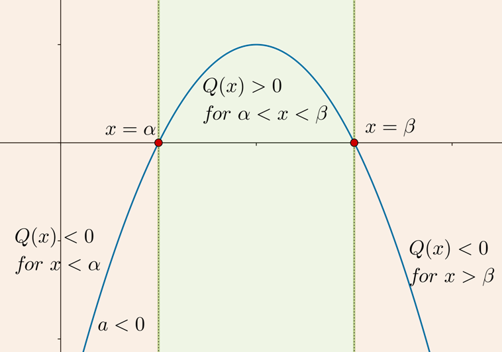Suppose that you are asked to find out all the values of x for which $${x^2} - 3x + 2 > 0$$. Let us first try an algebraic approach. By factorization, we can write the given inequality as

$\left( {x - 1} \right)\left( {x - 2} \right) > 0$

The product of two terms will be positive if both of them are positive, or both are negative. The two brackets on the left side above are both positive if $$x > 2$$, and are both negative if $$x < 1$$$$- {x^2} + 3x - 2 < 0$$. Thus, we can say that values of x which satisfy this inequality are $$x < 1$$ or $$x > 2$$.

A graphical approach is also possible. The graph of $$Q\left( x \right)\,\,:\,\,{x^2} - 3x + 2$$ is plotted below:Note the heavy parts of the curve. These lie above the horizontal axis, that is, they correspond to positive values of the expression. The corresponding x-values are $$x < 1$$ or $$x > 2$$.

Now, consider the quadratic inequality $${x^2} - 3x + 2 < 0$$. This can be written as

$\left( {x - 1} \right)\left( {x - 2} \right) < 0$

The product of two terms is negative if the two terms are of opposite signs. The two brackets on the left side above are of opposite signs if x is less than 2 but greater than 1. Thus, this inequality is satisfied if $$1 < x < 2$$. Graphically, this corresponds to the part of the parabola below the -axis:Next, consider the inequality . By multiplying with $$- 1$$ across both sides of the inequality, it changes to:

${x^2} - 3x + 2 > 0$

Note that the sign of the inequality has reversed, because we multiplied with a negative quantity on both sides. Now, it can be solved as done earlier to obtain the solution as $$x < 1$$ or $$x > 2$$.

Alternatively, if we plot the graph of $$Q\left( x \right)\,\,:\,\, - {x^2} + 3x - 2$$, we will obtain an inverted parabola, as shown below. We note that $$Q\left( x \right) > 0$$ when $$x < 1$$ or $$x > 2$$. This is the same answer we obtained algebraically.

To summarize, suppose that we have a quadratic expression $$Q\left( x \right)\,\,:\,\,a{x^2} + bx + c$$ whose zeroes are $$\alpha ,\,\,\beta$$.

If $$a > 0$$, then

$\begin{array}{l} Q\left( x \right) > 0\,\,{\rm{when}}\,\,x < \alpha \,\,{\rm{or}}\,\,x > \beta \\Q\left( x \right) < 0\,\,{\rm{when}}\,\,\alpha < x < \beta \end{array}$

If $$a < 0$$, then

$\begin{array}{l}Q\left( x \right) > 0\,\,{\rm{when}}\,\,\alpha < x < \beta \\Q\left( x \right) < 0\,\,{\rm{when}}\,\,x < \alpha \,\,{\rm{or}}\,\,x > \beta \end{array}$

Remembering the following diagrams will help:grade 10 | Questions Set 2
grade 10 | Questions Set 1